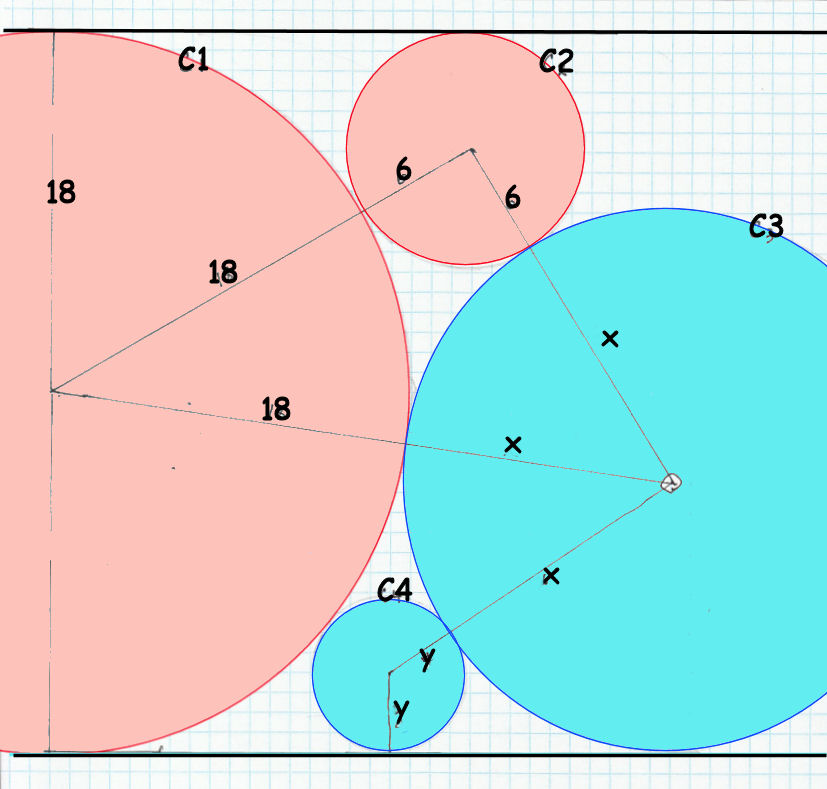# 2 Blue Moons

Geometry Level 54 circles are situated between 2 parallel lines and tangent to each other and to the lines as per diagram. The radii of $C_1$ and $C_2$ are 18 and 6 respectively..

If $x$ is the radius of $C_3$ and $y$ is the radius of $C_4$, find $x + y$ to the nearest 3 decimal places.

×